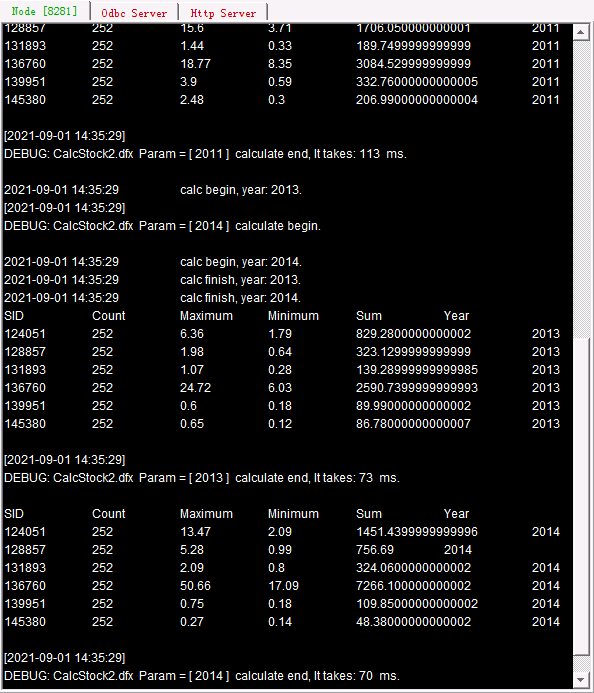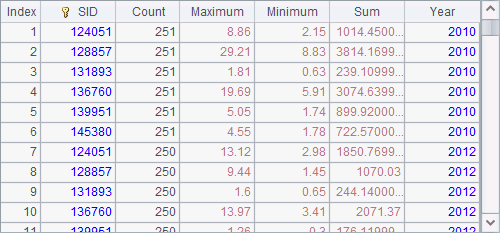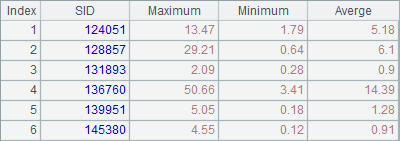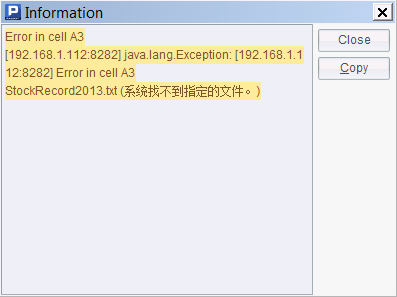# 坐标轴

## 数值轴

 A 1 =canvas() 2 =A1.plot("BackGround") 3 41 4 =A3.([round(pi(2*#-2)/(A3-1),3),round(sin(pi(2*#-2)/(A3-1)),3)]) 5 =A1.plot("NumericAxis","name":"x","autoCalcValueRange":false, "maxValue":7.0,"scaleNum":7,"xPosition":0.45) 6 =A1.plot("NumericAxis","name":"y","location":2,"autoCalcValueRange": false,"maxValue":1.0,"minValue":-1.0,"scaleNum":4) 7 =A1.plot("Line","markerStyle":0,"axis1":"x","data1":A4.(~(1)),"axis2":"y", "data2":A4.(~(2))) 8 =A1.draw@p(350,200)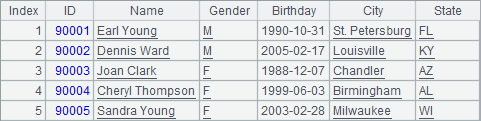A5中设置横轴，最大值为7，最小值为默认值0，同时将横轴绘制在纵轴的中间；A6中设置纵轴，最大值为1，最小值为-1A7中绘制折线图，折线图的逻辑坐标来源于A4绘图结果如下：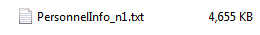## 日期轴

 A 1 =canvas() 2 =demo.query("select * from STOCKRECORDS where STOCKID='002242'") 3 =A1.plot("BackGround") 4 =A1.plot("DateAxis","name":"x","format":"dd/M","displayStep":20) 5 =A1.plot("NumericAxis","name":"y","location":2,"autoCalcValueRange": false,"maxValue":50.0) 6 =A1.plot("Line","markerWeight":2,"axis1":"x","data1":A2.(DATE),"axis2": "y","data2":A2.(CLOSING)) 7 =A1.draw@p(450,250)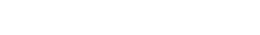A3绘制白色背景；A4中设定横轴x为日期轴，自动计算值域，且在刻度标签中仅显示交易日期；A5中设定纵轴y为数值轴，且最大值为50A6中绘制折线图，绘制的逻辑坐标为交易日期和收盘股价。A7绘制结果如下：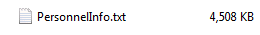## 枚举轴

 A 1 =canvas() 2 =demo.query("select * from FRUITS") 3 =A1.plot("BackGround") 4 =A1.plot("EnumAxis","name":"x","categories":A2.(NAME)) 5 =A1.plot("NumericAxis","name":"y","location":2) 6 =A1.plot("Column","axis1":"x","data1":A2.(NAME),"axis2":"y","data2": A2.(UNITPRICE)) 7 8 =A1.draw@p(350,200)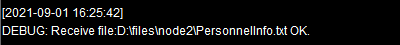A3绘制白色背景；A4中设定横轴x为枚举轴，并设定分类序列为水果名称构成的序列；A5中设定纵轴y为数值轴，自动计算值域；A6中绘制柱图，绘制的逻辑坐标为水果名称和水果单价。A8绘制结果如下：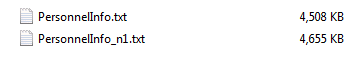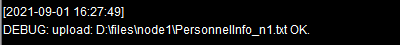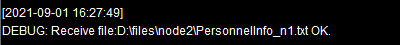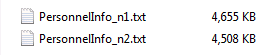A 1 =canvas() 2 =demo.query("select * from GYMSCORE") 3 =A1.plot("BackGround") 4 =A1.plot("EnumAxis","name":"x") 5 =A1.plot("NumericAxis","name":"y","location":2) 6 =A1.plot("Column","axis1":"x","data1":A2.(EVENT+","+NAME),"axis2":"y","data2":A2.(SCORE)) 7 8 =A1.draw@p(450,250)A3绘制白色背景；A4中设定横轴x为枚举轴，自动分析数据；A5中设置纵轴y为数值轴；A6中绘制柱图。同时使用分类和系列时，枚举轴上的逻辑坐标需要包含分类和系列的信息，将分类和系列的值用逗号分隔开，如"Vault,Becky Downie"

A8中绘图结果如下：## 极坐标系中的极轴和角轴

 A 1 =canvas() 2 =demo.query("select * from GYMSCORE where EVENT = 'Vault'") 3 =A1.plot("BackGround") 4 =A1.plot("EnumAxis","name":"x","location":3,"polarX":0.5,"allowLabels":false) 5 =A1.plot("NumericAxis","name":"y","location":4,"allowLabels":false) 6 =A1.plot("Sector","text":A2.(NAME),"axis1":"x","data1":A2.(NAME), "axis2":"y","data2":A2.(SCORE)) 7 =A1.draw@p(450,350)A4中设定极轴x为枚举轴，并选择不显示标签，并设定极点的物理横坐标为0.5，居中绘制。A5中设定角轴y为数值轴，同样不显示标签，而且并不修改角轴相关属性的默认值：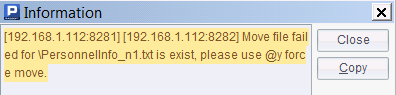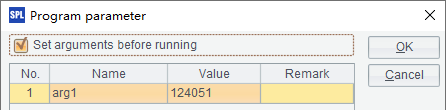A6中绘制饼图，绘制的逻辑坐标为运动员姓名和得分，并标注运动员姓名。A7绘制结果如下：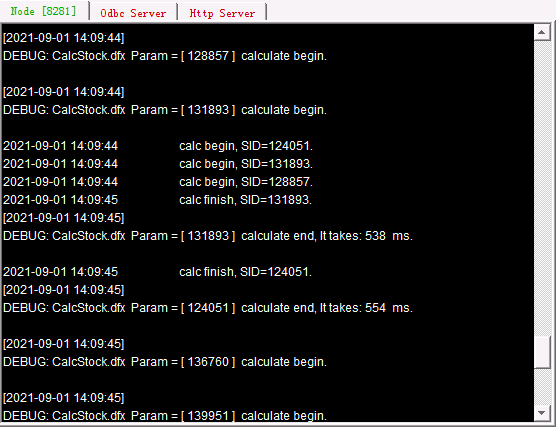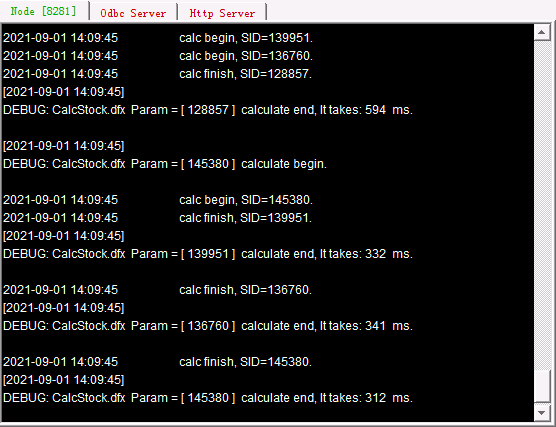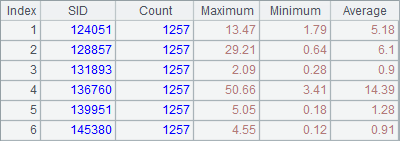## 图例

 A 1 =canvas() 2 =demo.query("select * from FRUITS") 3 =A1.plot("BackGround") 4 =A1.plot("EnumAxis","name":"x","categories":A2.(NAME)) 5 =A1.plot("NumericAxis","name":"y","location":2) 6 =A1.plot("Column","axis1":"x","data1":A2.(NAME),"axis2":"y","data2": A2.(UNITPRICE)) 7 =A1.plot("Legend","legendText":A2.(NAME),"x":0.81,"y":0.2,"width":0.18) 8 =A1.draw@p(350,200)

A7中添加了图例图元的代码，设定了图例数据及其位置如下：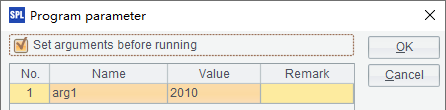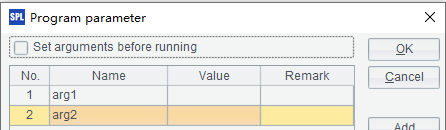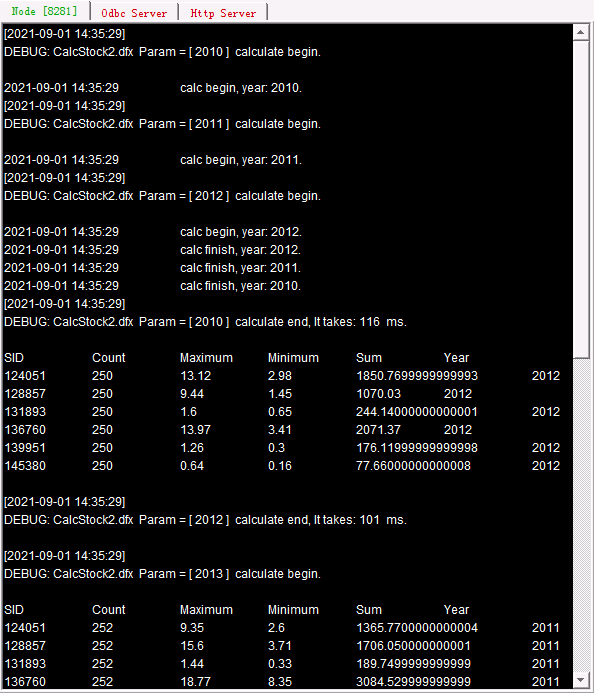A 1 =canvas() 2 =demo.query("select * from GYMSCORE") 3 =A1.plot("BackGround") 4 =A1.plot("EnumAxis","name":"x") 5 =A1.plot("NumericAxis","name":"y","location":2) 6 =A1.plot("Column","axis1":"x","data1":A2.(EVENT+","+NAME),"axis2":"y","data2":A2.(SCORE)) 7 =A1.plot("Legend","legendText":A2.id(NAME),"x":0.81,"y":0.2,"width": 0.18) 8 =A1.draw@p(450,250)

A7中添加了图例图元的代码，设定了图例数据及其位置如下：